# Viscosity corrections to pump curve

Viscosity corrections to the pump curve 14-04

Viscosity means resistance to pouring. The higher the viscosity the thicker the fluid. If you are not familiar with the term viscous fluid please refer to my paper Volume 7 , Number 8. Here is what is going to change when you pump viscous fluids with a centrifugal pump:

• The brake horsepower requirement will increase.
• You will notice a reduction in the head the pump will produce.
• Some reduction in capacity will occur with moderate and high viscosities.
• The pump’s efficiency will decrease.

The attached chart is typical of the charts supplied by the hydraulic Institute and other similar publications. You can use it to:

• Act as an aid in selecting the pump you need for your application.
• Determine the performance of a conventional centrifugal pump pumping a viscous fluid when its performance with only water is known.

In this section we will address the first use of the chart and let it assist us in selecting the centrifugal pump for viscous fluids. Centrifugal pumps have been used with viscosities below 3,000 SSU (660 centistokes) and as high as 15,000 SSU (3,300 centistokes) but they are sensitive to changing viscosity.

High viscosity fluids are better handled with positive displacement pumps that are affected differently than centrifugal pumps by a change in fluid viscosity:

• At a constant speed, changes in viscosity will have very little affect on capacity.
• The total head will probably increase with viscosity because of higher system resistance.
• The brake horsepower (kilowatts) will increase with capacity.
• The efficiency probably will not be affected because of less leakage through the internal pump clearances. In some cases the efficiency will incease

Viscous fluids are very common in the petroleum industry so the attached chart was prepared using petroleum oils as the test fluid, and like all charts of this type the information is general.

If you need very accurate information you will use this chart only as a guide and then conduct your own test with your specific fluid. This information will get you into the “ball park”. Use the chart only for:

• Conventional centrifugal pump hydraulic designs only.
• Pumps operating close to their BEP, and not at the far ends of the pump curve.
• Pumps operating with enough net positive suction head available (NPSHA) to prevent cavitation.
• Newtonian fluids. These are the type that do not change their viscosity with agitation. Non-Newtonian fluids can increase or decrease their viscosity with agitation. As an example if you agitate cream it will increase in viscosity and become butter.
• The information in the chart and formulas is generally valid for both open and closed impellers.

We will be using the following conventional symbols in our formulas with the exception of the “N” for efficiency. The efficiency symbol used in most formulas is a Greek letter and looks a little different, but this is the best I can do at this time, so bear with me:

Qvis Viscous capacity in gpm. (m3/hr). The capacity when pumping a viscous fluidHvis Viscous head in feet (meters). The head when pumping a viscous fluid.

Nvis Viscous efficiency in percent. The efficiency when pumping a viscous fluid

bhpvis Viscous brake horse power. The horsepower ( Kilowatts) required by the pump for the viscous conditions.

Qw Water capacity in gpm.(m3/hr) The capacity when pumping water.

Nw Water efficiency in percent. The efficiency when pumping water.

S Specific gravity.

Cq Capacity correction factor.

Cn Efficiency correction factor.

Qnw Water capacity at which maximum efficiency is obtained

The following equations are used for determining the viscous performance when the water performance of the pump is known. As you can see they are not very hard to understand or calculate once you get the terms straight.Cq , Ch and Cn are determined from the correction chart which is based on water performance.On the chart they are shown as CQ, CH and CN

The following equations are used for approximating the water performance when the desired viscous capacity and head are given and the values of Cq and Ch must be estimated from the chart using Qvis and Hvis as :If you are not comfortable working with formulas all of these numbers look real scary, but the formulas are really quite basic and require nothing more than the ability to multiply and divide simple numbers.

Almost every calculation in the pump and seal business can be done with a low cost hand calculator. So jump right in and get wet.

With all of that said, we will use this information to select a pump for a given head-capacity-viscosity condition. The first two examples will be in inch units and then we will show an example in metric units.

We are looking for a pump to pump 750 gpm to a total head (this includes the static, pressure and friction heads combined) of 100 feet with a liquid having a viscosity of 1000 SSU and a specific gravity of 0.90 at the pumping temperature.

Please take a look at the following chart:At the bottom of the chart enter at 750 go up to 100 feet, over to 1000 SSU and then up to the correction factors shown below :

• Cq = 0.95
• Ch = 0.92 (for 1.0 Qnw water capacity))
• Cn = 0.635
• Qw = 750/0.95 = 790 gpm (see the last formulas shown above)
• Hw = 100/0.92 = 108.8 or 109 feet head (see the formula above)
• = 0.90

This means that you need a pump with a water capacity of 790 gpm. At a head of 109 feet.

If the pump selected has an efficiency of 81% when it is pumping water the efficiency pumping the viscous fluid will drop to Nvis = 0.635 x 81% = 51.5 percent efficient.

The brake horsepower for the viscous fluid will become: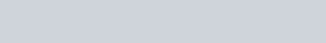That wasn’t too bad was it? Here is another example. See if you come up with similar results. This time the pump is going to handle 500 gpm (114 m3/hr) of 3000 SSU (660 centistokes) liquid against a head of 150 feet (46 meters) Looking at the chart :

Cq = 0.80 and Ch = 0.81

The water capacity becomes:

• In USCS units Qw = 500/0.80 = 625 gpm
• In SI units Qw = 114/0.80 = 142 m3/hr

• In USCS Qh = 150/0.81 = 185 ft.
• In SI units Qh = 46/0.81 = 57 meters

Going to the graph for 625 gpm (142 m3/hr), 185 feet (57 meters), 3000 SSU (660 cSt), the graph shows Cq = 0.83

Adjusting the water capacity we get

• Qw x (0.80/0.83) = 602 gpm or 137m3/hr.

• Hw x (0.81/0.84) = 178 feet (55 meters)

The efficiency will drop to Nvis = 0.75 x 42% = 31.5 percent

• In USCS units the brake horsepower for the viscous fluid will become: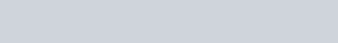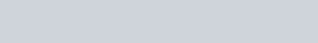• In SI units the brake kilowatts for the viscous fluid will become: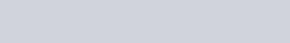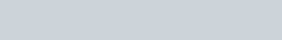### Posted

• On February 18, 2018